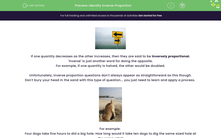# Identify Inverse Proportion

In this worksheet, students will explore relationships which are inversely proportional, using information provided to find the value of a single unit, then applying this information to a new total to solve a real-world problem.Key stage:  KS 4

Year:  GCSE

GCSE Subjects:   Maths

GCSE Boards:   AQA, Eduqas, Pearson Edexcel, OCR,

Curriculum topic:   Ratio, Proportion and Rates of Change

Curriculum subtopic:   Ratio, Proportion and Rates of Change Direct and Inverse Proportion

Difficulty level:#### Worksheet OverviewIf one quantity decreases as the other increases, then they are said to be inversely proportional.

'Inverse' is just another word for doing the opposite.

For example, if one quantity is halved, the other would be doubled.

Unfortunately, inverse proportion questions don't always appear as straightforward as this though.

Don't bury your head in the sand with this type of question... you just need to learn and apply a process.For example:

Four dogs take five hours to did a big hole. How long would it take ten dogs to dig the same sized hole at the same rate?

Let's make a table to summarise what we know and what we want to find out:

 4 dogsTake 5 hours 1 dog 5 × 4 20 hours 10 dogs 20 ÷ 10 2 hours

So we used what we know to find the corresponding amount for a single unit (in this case 'one dog'), then applied this to the new total.

Let's try another problem to practise this process more.For example:

It takes 5 bakers, 4 hours to bake 125 cakes. How long would it take 8 bakers to make 200 cakes if they all work at the same rate?

 5 bakersTake 4 hours 1 baker 4 × 5 20 hours 8 bakers 20 ÷ 8 2.5 hours (for 125 cakes) 1 cake 2.5 ÷ 125 0.02 hours 200 cakes 0.02 × 100 4 hours

Don't you think it is unfair that you always have to do that bit extra before you can get some cake?

Now let's put this process into action!

In this activity, we will explore relationships which are inversely proportional, using the information provided to find the value of a single unit, then applying this information to a new total to solve a real-world problem.

### What is EdPlace?

We're your National Curriculum aligned online education content provider helping each child succeed in English, maths and science from year 1 to GCSE. With an EdPlace account you’ll be able to track and measure progress, helping each child achieve their best. We build confidence and attainment by personalising each child’s learning at a level that suits them.

Get started